• MIOU定义 有很多博客都有写MIOU，参考下面文章 注意点： 类别包含背景，二分类也包含背景 混淆矩阵就是分别统计分类模型归错类，归对类的观测值个数，然后把结果放在一个表里展示出来。 代码实现 def fast_hist(a,...
MIOU定义
有很多博客都有写MIOU，参考下面文章 注意点：
类别包含背景，二分类也包含背景混淆矩阵就是分别统计分类模型归错类，归对类的观测值个数，然后把结果放在一个表里展示出来。
代码实现
def fast_hist(a, b, n):
'''
a是W*H的一维数组，预测值
b是W*H的一维数组，真实值
n是类别数，包含背景，二分类时n=2
'''
# k是一个一维bool数组，形状(H×W,)；目的是找出标签中需要计算的类别
k = (a >= 0) & (a < n)
# np.bincount计算了从0到n**2-1这n**2个数中每个数出现的次数，返回值形状(n, n)
# 计算例子参考下面博客，虽然例子有错误地方，不影响理解代码
return np.bincount(n * a[k].astype(int) + b[k], minlength=n ** 2).reshape(n,n)）
def per_class_iu(hist):  # 分别为每个类别（在这里是19类）计算mIoU，hist的形状(n, n)
'''
计算MIOU
'''
# 矩阵的对角线上的值组成的一维数组/矩阵的所有元素之和，返回值形状(n,)
return np.diag(hist) / (hist.sum(1) + hist.sum(0) - np.diag(hist))

参考博客
：语义分割之MIoU原理与实现 ：4.4.2分类模型评判指标（一） - 混淆矩阵(Confusion Matrix)
展开全文深度学习
• 1、MIOU定义 Mean Intersection over Union(MIoU，均交并比)：为语义分割的标准度量。其计算两个集合的交集和并集之比，在语义分割的问题中，这两个集合为真实值和预测值。在每个类上计算IoU，之后平均。计算公式...
1、MIOU定义
Mean Intersection over Union(MIoU，均交并比)：为语义分割的标准度量。其计算两个集合的交集和并集之比，在语义分割的问题中，这两个集合为真实值和预测值。在每个类上计算IoU，之后平均。计算公式如下：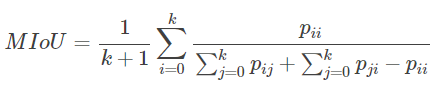i表示真实值，j表示预测值 ，p_{ij}pij​ 表示将i预测为j 。

2、直观理解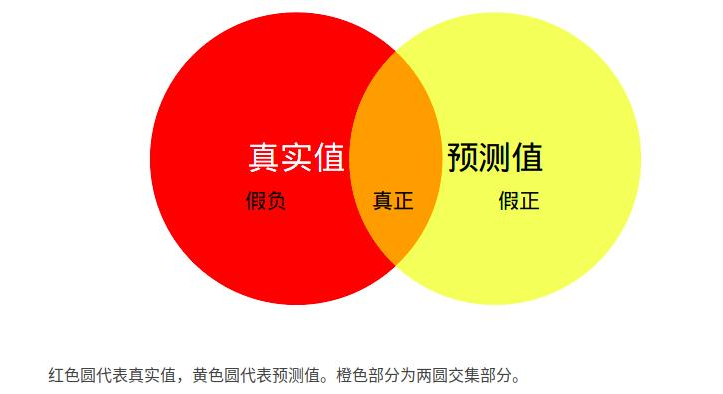MIoU：计算两圆交集（橙色部分）与两圆并集（红色+橙色+黄色）之间的比例，理想情况下两圆重合，比例为1。

3、MIOU实现
（1）先求混淆矩阵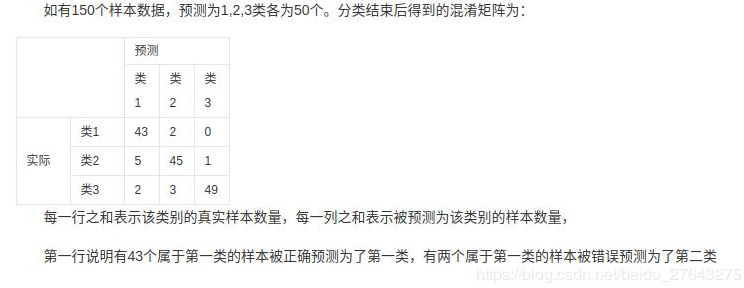（2）再求mIOU
混淆矩阵的每一行再加上每一列，最后减去对角线上的值
import numpy as np
class IOUMetric:     """     Class to calculate mean-iou using fast_hist method     """
def __init__(self, num_classes):         self.num_classes = num_classes         self.hist = np.zeros((num_classes, num_classes))
def _fast_hist(self, label_pred, label_true):         # 找出标签中需要计算的类别,去掉了背景         mask = (label_true >= 0) & (label_true < self.num_classes)         # # np.bincount计算了从0到n**2-1这n**2个数中每个数出现的次数，返回值形状(n, n)         hist = np.bincount(             self.num_classes * label_true[mask].astype(int) +             label_pred[mask], minlength=self.num_classes ** 2).reshape(self.num_classes, self.num_classes)         return hist
# 输入：预测值和真实值     # 语义分割的任务是为每个像素点分配一个label     def ev aluate(self, predictions, gts):         for lp, lt in zip(predictions, gts):             assert len(lp.flatten()) == len(lt.flatten())             self.hist += self._fast_hist(lp.flatten(), lt.flatten())                      # miou         iou = np.diag(self.hist) / (self.hist.sum(axis=1) + self.hist.sum(axis=0) - np.diag(self.hist))         miou = np.nanmean(iou)                   # -----------------其他指标------------------------------         # mean acc         acc = np.diag(self.hist).sum() / self.hist.sum()         acc_cls = np.nanmean(np.diag(self.hist) / self.hist.sum(axis=1))
freq = self.hist.sum(axis=1) / self.hist.sum()         fwavacc = (freq[freq > 0] * iou[freq > 0]).sum()
return acc, acc_cls, iou, miou, fwavacc  
展开全文• 直观理解 MIoU：计算两圆交集（橙色部分）与两圆并集（红色+橙色+黄色）之间的比例，理想情况下两圆重合，比例为1。 Reference 【语义分割】语义分割评估指标mIOU ...
直观理解
MIoU：计算两圆交集（橙色部分）与两圆并集（红色+橙色+黄色）之间的比例，理想情况下两圆重合，比例为1。Reference
【语义分割】语义分割评估指标mIOU
展开全文• mIOU定义 Mean Intersection over Union(MIoU，均交并比)，为语义分割的标准度量。其计算两个集合的交集和并集之比，在语义分割问题中，这两个集合为真实值（ground truth）和预测值（predicted segmentation）。这...
mIOU定义
Mean Intersection over Union(MIoU，均交并比)，为语义分割的标准度量。其计算两个集合的交集和并集之比，在语义分割问题中，这两个集合为真实值（ground truth）和预测值（predicted segmentation）。这个比例可以变形为TP（交集）比上TP、FP、FN之和（并集）。在每个类上计算IoU，然后取平均。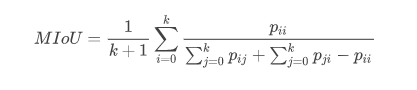pij表示真实值为i，被预测为j的数量。 等同于：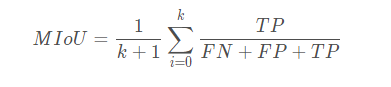直观理解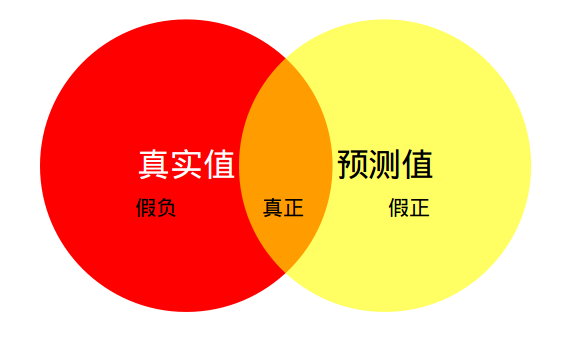红色圆代表真实值，黄色圆代表预测值。橙色部分为两圆交集部分。
MPA（Mean Pixel Accuracy，均像素精度）：计算橙色与红色圆的比例； MIoU：计算两圆交集（橙色部分）与两圆并集（红色+橙色+黄色）之间的比例，理想情况下两圆重合，比例为1。
步骤一：先求混淆矩阵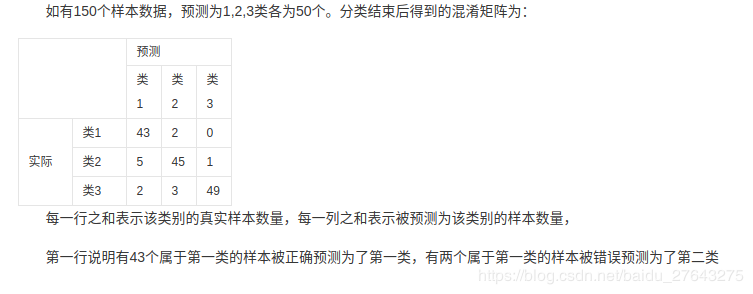步骤二：再求mIOU
Pytorch实现
import numpy as np

class IOUMetric:
"""
Class to calculate mean-iou using fast_hist method
"""

def __init__(self, num_classes):
self.num_classes = num_classes
self.hist = np.zeros((num_classes, num_classes))

def _fast_hist(self, label_pred, label_true):
# 找出标签中需要计算的类别,去掉了背景
mask = (label_true >= 0) & (label_true < self.num_classes)
# np.bincount计算了从0到n**2-1这n**2个数中每个数出现的次数，返回值形状(n, n)
hist = np.bincount(
return hist

# 输入：预测值和真实值
# 语义分割的任务是为每个像素点分配一个label
def evaluate(self, predictions, gts):
for lp, lt in zip(predictions, gts):
self.hist += self._fast_hist(lp.flatten(), lt.flatten())
#miou
iou = np.diag(self.hist) / (self.hist.sum(axis=1) + self.hist.sum(axis=0) - np.diag(self.hist))
miou = np.nanmean(iu)
#其他性能指标
acc = np.diag(self.hist).sum() / self.hist.sum()
acc_cls = np.nanmean(np.diag(self.hist) / self.hist.sum(axis=1))
freq = self.hist.sum(axis=1) / self.hist.sum()
fwavacc = (freq[freq > 0] * iu[freq > 0]).sum()
return acc, acc_cls, iou, miou, fwavacc

参考：mIoU源码解析
展开全文• 语义分割评价指标mIOU的计算 ## 注意事项 这是针对重构了的语义分割网络，而不是之前的那个，所以不要询问原来的网络计算miou要怎么做，因为整个文件构架差距过大，建议使用新构架。 学习前言 算一下语义分割的miou...深度学习
• 憨批的语义分割10——语义分割评价指标mIOU的计算学习前言什么是mIOUPSPNet实现思路一、预测部分1、主干网络介绍2、加强特征提取结构3、利用特征获得预测结果二、训练部分1、训练文件详解2、LOSS解析训练自己的...机器学习 深度学习 语义分割
• 深度学习计算机视觉图像分割领域指标mIoU（平均交并比）计算代码与逐行解析mIoU相关mIoU计算代码与逐行解析注意事项 mIoU相关 在计算机视觉深度学习图像分割领域中，mIoU值是一个衡量图像分割精度的重要指标mIoU...图像分割 计算机视觉 深度学习
• 语义分割评估指标计算公式可参考：基于深度学习的图像语义分割技术概述之5.1度量标准 mIOU定义 Mean Intersection over Union(MIoU，均交并比)：为语义分割的标准度量。其计算两个集合的交集和并集之比，在语义分割...
• 常用的评价指标 在评价的时候常用的指标有：IOU（交并比，也有叫做IU的），像素准确率（pixel-accuracy），有的时候还有平均准确率（mean-accuracy）。 1.IoU or IU（intersection over union） IoU指标就是大家常...
• 语义分割mIOU定义如下：   其中p_ij表示真实值为i，被预测为j的像素数量，等价于   其中TP(t)表示以为阈值的情况下预测为正样本，实际上也为正样本的数量。 Dice coefficient定义如下：
• Pixel Accuracy(PA，像素精度)：这是最简单的度量，为标记正确的像素占总像素的比例。...Mean Intersection over Union(MIoU，均交并比)：为语义分割的标准度量。其计算两个集合的交集和并集之比，在语义分割的...
• 一、通过histogram计算miou、precision、recall 通过python的numpy包中的histogram函数计算图像语义分割的评价指标。 这里想得出每一类的评价指标，而不是一个整体的，故采用 对于某一张图片 imlabel和impred均为h*w...
• 于是，我就找了目前几个主流的评测指标如下： Pixel Accuracy(PA，像素精度)：这是最简单的度量，为标记正确的像素占总像素的比例。 Mean Pixel Accuracy(MPA，均像素精度)：是PA的一种简单提升，计算每个...深度学习 语义分割
• 【语义分割】评价指标：PA、CPA、MPA、IoU、MIoU详细总结和代码实现（零基础从入门到精通系列！） 本文主要是想写一个用Pytorch计算的方法。当初想着直接拿这些评价指标的倒数作为loss来训练网络，所以才想着用...pytorch 深度学习 机器学习
• # 计算并写出precision，recall, f1-score，f1-m以及mIOU f1_m = [] iou_m = [] for i in range(class_num): # 写出f1-score f1 = TP[i] * 2 / (TP[i] * 2 + FP[i] + FN[i]) f1_m.append(f1) iou = TP[i] /...
• 语义分割，简单地讲就是给一张图像，分割分出一个物体的准确轮廓。其实就是分类任务，而分类任务预测的结果往往就是一下四种： TP：True Positive FP：False Positive TN... MIoU = A∩B / A∪B = TP / (FP + FN + TP)计算机视觉 人工智能
• # -----------------其他指标------------------------------ # mean acc acc = np.diag(self.hist).sum() / self.hist.sum() acc_cls = np.nanmean(np.diag(self.hist) / self.hist.sum(axis=1)) freq = self...
• 图像分割评价指标---mIoU（平均交并比）计算代码（OpenCV/C++） 运行环境：Visual Studio+OpenCV+C++ 输入：准备好的Groundtruth数据+分割得到的二值图像 输出：每张分割结果的IOU值+最后输出MIOU值（平均交并比） ...OpenCV C++ 平均交并比 代码
• 图像分割评价指标之FWIoU图像分割评价指标之FWIoU图像分割评价指标之FWIoU...